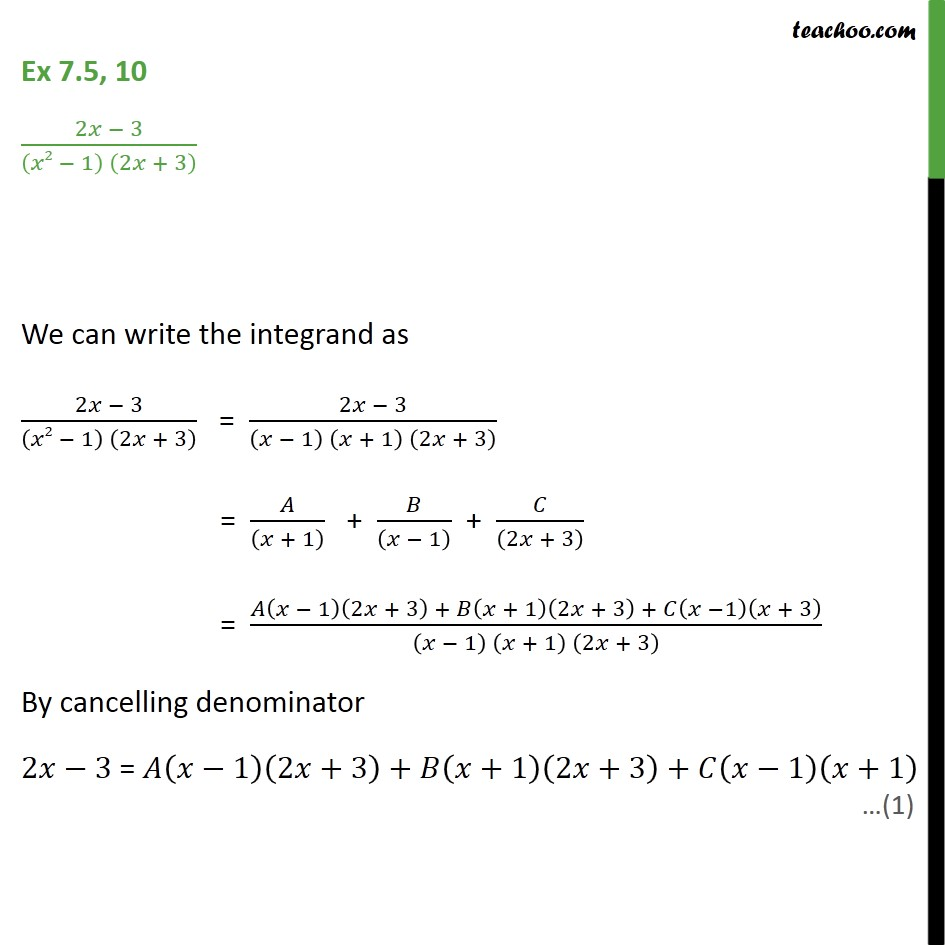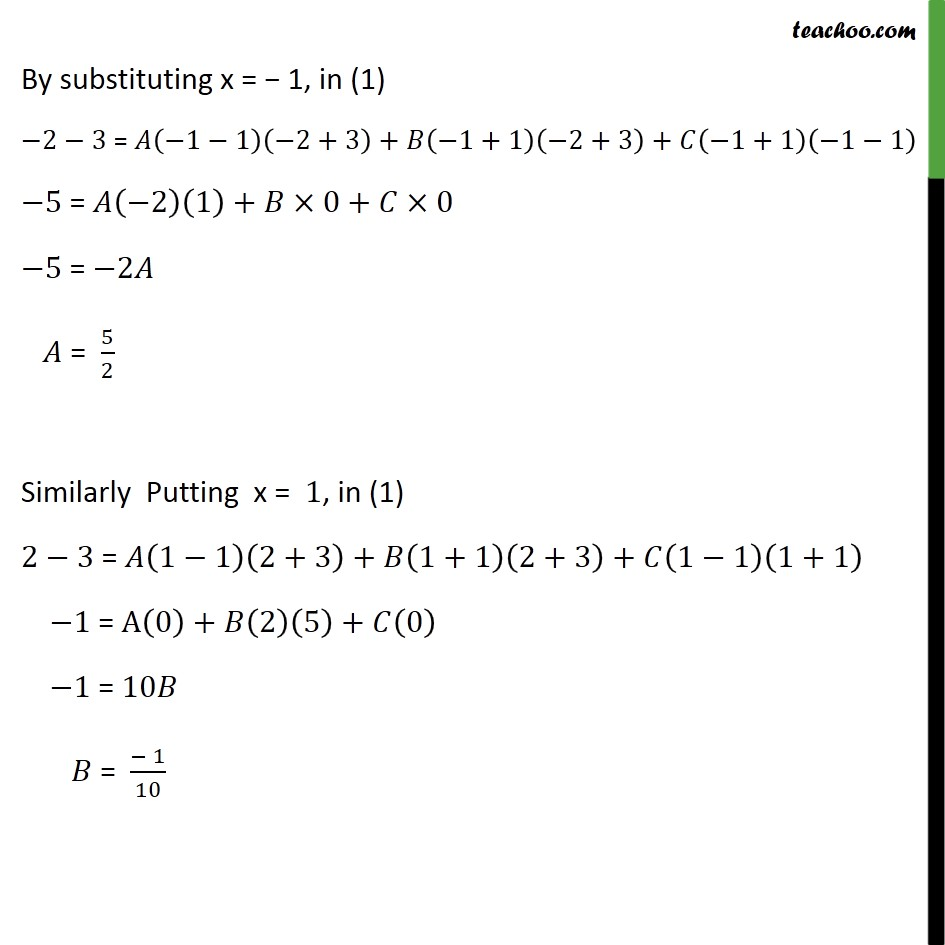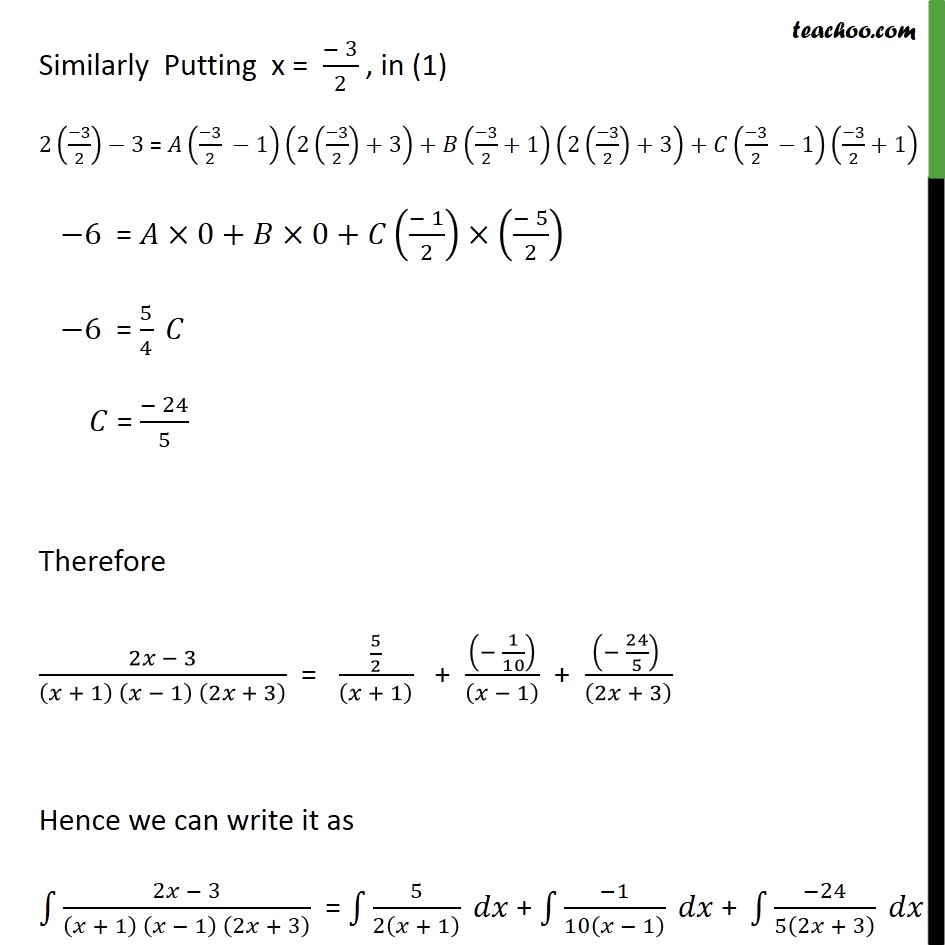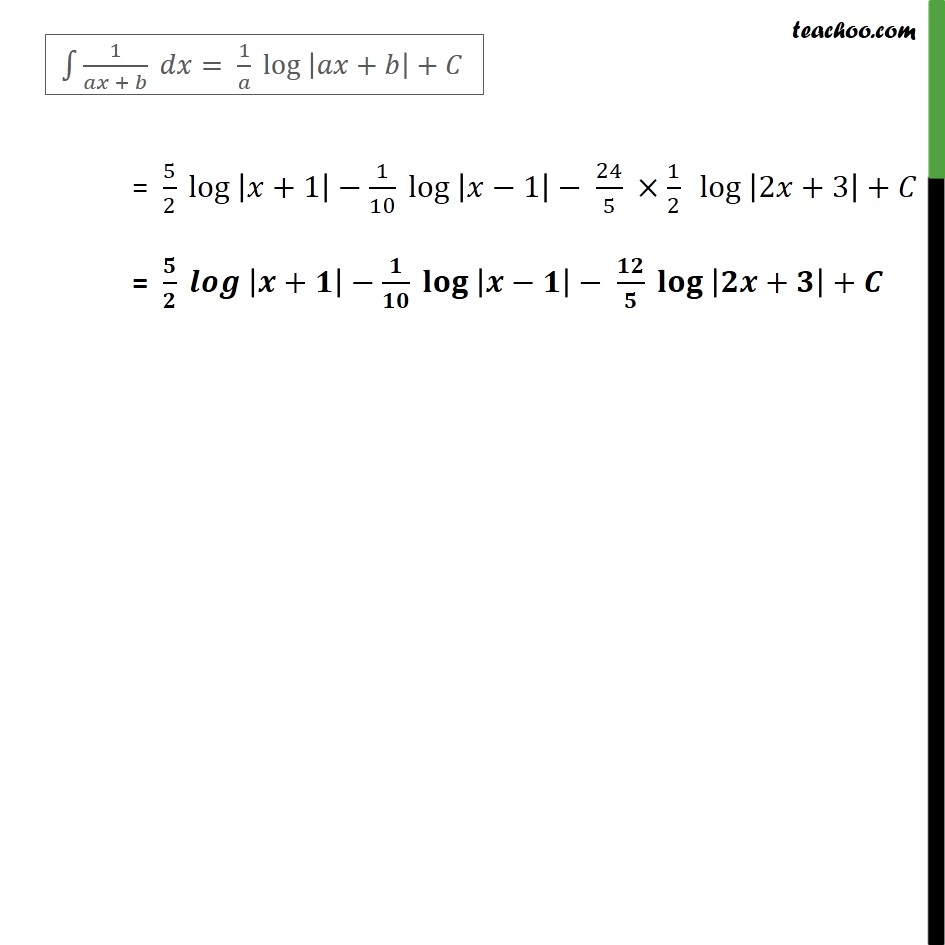Integration by partial fraction - Type 3

Chapter 7 Class 12 Integrals
Concept wiseIntroducing your new favourite teacher - Teachoo Black, at only ₹83 per month

### Transcript

Ex 7.5, 10 2 3 2 1 2 + 3 We can write the integrand as 2 3 2 1 2 + 3 = 2 3 1 + 1 2 + 3 = + 1 + 1 + 2 + 3 = 1 2 + 3 + + 1 2 + 3 + 1 + 3 1 + 1 2 + 3 By cancelling denominator 2 3 = 1 2 +3 + +1 2 +3 + 1 +1 By substituting x = 1, in (1) 2 3 = 1 1 2+3 + 1+1 2+3 + 1+1 1 1 5 = 2 1 + 0+ 0 5 = 2 = 5 2 Similarly Putting x = 1, in (1) 2 3 = 1 1 2+3 + 1+1 2+3 + 1 1 1+1 1 = A 0 + 2 5 + 0 1 = 10 = 1 10 Similarly Putting x = 3 2 , in (1) 2 3 2 3 = 3 2 1 2 3 2 +3 + 3 2 +1 2 3 2 +3 + 3 2 1 3 2 +1 6 = 0+ 0+ 1 2 5 2 6 = 5 4 = 24 5 Therefore 2 3 + 1 1 2 + 3 = 5 2 + 1 + 1 10 1 + 24 5 2 + 3 Hence we can write it as 2 3 + 1 1 2 + 3 = 5 2 + 1 + 1 10 1 + 24 5 2 + 3 = 5 2 log +1 1 10 log 1 24 5 1 2 log 2 +3 + = + + +